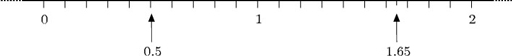Numbers, units and arithmetic

This free course is available to start right now. Review the full course description and key learning outcomes and create an account and enrol if you want a free statement of participation.

Free course

# 1.5 Decimals

Quantities can be smaller than one (such as 0.5 kg) or take values between whole numbers (such as a height of 1.65 metres). Numbers smaller than one are expressed as decimals or as fractions. Decimals are often easier to work with (especially when using a calculator). Decimals are explained in this section, and fractions following that (Section 1.7).

Decimals can be indicated on the number line in between whole numbers. 0.5 and 1.65 are indicated on the figure below.Decimals arise when whole numbers are divided by ten, a hundred etc.

MU120_4M1